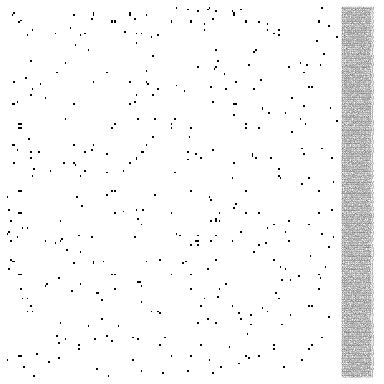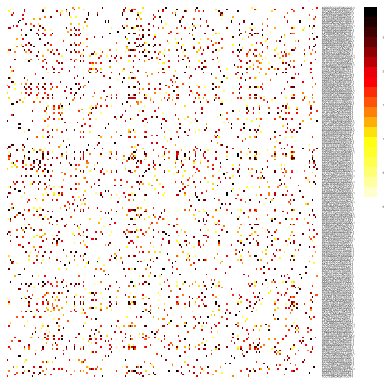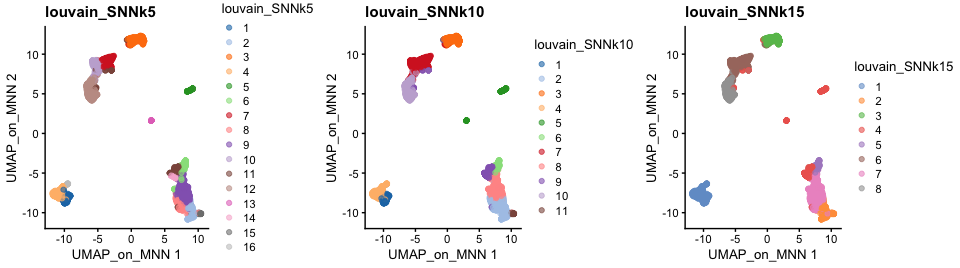# Clustering

In this tutorial we will continue the analysis of the integrated dataset. We will use the integrated PCA to perform the clustering. First we will construct a \(k\)-nearest neighbour graph in order to perform a clustering on the graph. We will also show how to perform hierarchical clustering and k-means clustering on PCA space.

Let’s first load all necessary libraries and also the integrated dataset from the previous step.

``````suppressPackageStartupMessages({
library(scater)
library(scran)
library(cowplot)
library(ggplot2)
library(rafalib)
library(pheatmap)
library(igraph)
})

## Graph clustering

The procedure of clustering on a Graph can be generalized as 3 main steps:

1. Build a kNN graph from the data

2. Prune spurious connections from kNN graph (optional step). This is a SNN graph.

3. Find groups of cells that maximizes the connections within the group compared other groups.

### Building kNN / SNN graph

The first step into graph clustering is to construct a k-nn graph, in case you don’t have one. For this, we will use the PCA space. Thus, as done for dimensionality reduction, we will use ony the top N PCA dimensions for this purpose (the same used for computing UMAP / tSNE).

``````# These 2 lines are for demonstration purposes only
g <- buildKNNGraph(sce, k = 30, use.dimred = "MNN")

# These 2 lines are the most recommended
g <- buildSNNGraph(sce, k = 30, use.dimred = "MNN")
reducedDim(sce, "SNN") <- as_adjacency_matrix(g, attr = "weight")``````

We can take a look at the kNN graph. It is a matrix where every connection between cells is represented as \(1\)s. This is called a unweighted graph (default in Seurat). Some cell connections can however have more importance than others, in that case the scale of the graph from \(0\) to a maximum distance. Usually, the smaller the distance, the closer two points are, and stronger is their connection. This is called a weighted graph. Both weighted and unweighted graphs are suitable for clustering, but clustering on unweighted graphs is faster for large datasets (> 100k cells).

``````# plot the KNN graph
pheatmap(reducedDim(sce, "KNN")[1:200, 1:200], col = c("white", "black"), border_color = "grey90",
legend = F, cluster_rows = F, cluster_cols = F, fontsize = 2)````````````# or the SNN graph
pheatmap(reducedDim(sce, "SNN")[1:200, 1:200], col = colorRampPalette(c("white",
"yellow", "red", "black"))(20), border_color = "grey90", legend = T, cluster_rows = F,
cluster_cols = F, fontsize = 2)``````As you can see, the way Scran computes the SNN graph is different to Seurat. It gives edges to all cells that shares a neighbor, but weights the edges by how similar the neighbors are. Hence, the SNN graph has more edges than the KNN graph.

### Clustering on a graph

Once the graph is built, we can now perform graph clustering. The clustering is done respective to a resolution which can be interpreted as how coarse you want your cluster to be. Higher resolution means higher number of clusters.

``````g <- buildSNNGraph(sce, k = 5, use.dimred = "MNN")
sce\$louvain_SNNk5 <- factor(cluster_louvain(g)\$membership)

g <- buildSNNGraph(sce, k = 10, use.dimred = "MNN")
sce\$louvain_SNNk10 <- factor(cluster_louvain(g)\$membership)

g <- buildSNNGraph(sce, k = 15, use.dimred = "MNN")
sce\$louvain_SNNk15 <- factor(cluster_louvain(g)\$membership)

plot_grid(ncol = 3, plotReducedDim(sce, dimred = "UMAP_on_MNN", colour_by = "louvain_SNNk5") +
ggplot2::ggtitle(label = "louvain_SNNk5"), plotReducedDim(sce, dimred = "UMAP_on_MNN",
colour_by = "louvain_SNNk10") + ggplot2::ggtitle(label = "louvain_SNNk10"), plotReducedDim(sce,
dimred = "UMAP_on_MNN", colour_by = "louvain_SNNk15") + ggplot2::ggtitle(label = "louvain_SNNk15"))``````We can now use the `clustree` package to visualize how cells are distributed between clusters depending on resolution.

``````suppressPackageStartupMessages(library(clustree))

clustree(sce, prefix = "louvain_SNNk")``````